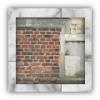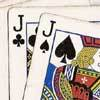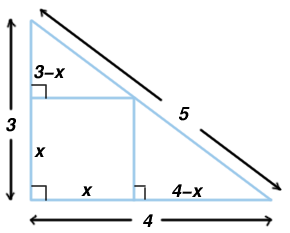#### You may also like### Rule of Three

If it takes four men one day to build a wall, how long does it take 60,000 men to build a similar wall?### Square Pegs

Which is a better fit, a square peg in a round hole or a round peg in a square hole?### Do Unto Caesar

At the beginning of the night three poker players; Alan, Bernie and Craig had money in the ratios 7 : 6 : 5. At the end of the night the ratio was 6 : 5 : 4. One of them won \$1 200. What were the assets of the players at the beginning of the evening?

# How Big?

##### Age 11 to 14Challenge Level

The following solution came from Alistair Jacklin, class 9S, King Edward Camp Hill School for Boys. Kang Hong Joo of The Chinese High School, Singapore and Neil Wright of Madras College, St Andrew's and Jessica Zhang, Woodbridge School, Essex used the same method.The first thing I did was to split the large triangle into 3 sections, a square and 2 smaller triangles. Next I then worked out the area of the large triangle using the formula:-

 Height * Width * 0.5 = area of the triangle 3 * 4 * 0.5 = area of a triangle 6 = area of a large triangle

With this I used a formula to show the area of this triangle by adding the areas of the other parts:

x 2 + 0.5 x(3 - x) + 0.5 x(4 - x) = 6.

The formula is then simplified in several easy steps to give:-

 x 2 + 1.5 x - 0.5 x 2 + 2 x - 0.5 x 2 = 6 3.5 x = 6 x = 12/7

The area of the square is then x 2 = 144/49 exactly. Rounded to three decimal places this is 2.938.